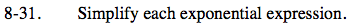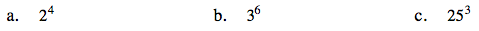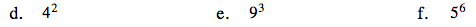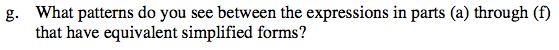Home > ACC7 > Chapter cc38 > Lesson cc38.1.3 > Problem8-31

8-31.

Simplify each exponential expression. Homework Help ✎

 a. 24 b. 36 c. 253 d. 42 e. 93 f. 56 g. What patterns do you see between the expressions in parts (a) through (f) whose simplified forms are equal?2 (2) (2) (2)

Multiply 3 by itself 6 times.

3 (3) (3) (3) (3) (3)

1562516

9 (9) (9)

729

Multiply 5 by itself 6 times.(a) and (d), (b) and (e), (c) and (f) have the same answers. How is this possible?

Notice that the base in (d) is the square of the base in (a).
How does this apply to the other two pairs?